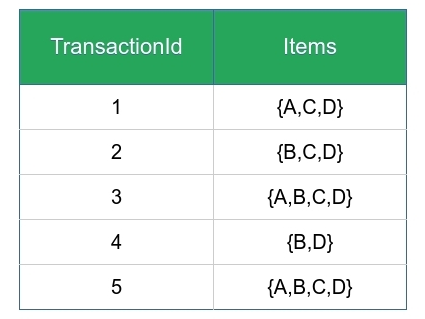Skip to content
Related Articles
Frequent Item set in Data set (Association Rule Mining)
• Difficulty Level : Hard
• Last Updated : 02 Mar, 2020

Association Mining searches for frequent items in the data-set. In frequent mining usually the interesting associations and correlations between item sets in transactional and relational databases are found. In short, Frequent Mining shows which items appear together in a transaction or relation.

Need of Association Mining:
Frequent mining is generation of association rules from a Transactional Dataset. If there are 2 items X and Y purchased frequently then its good to put them together in stores or provide some discount offer on one item on purchase of other item. This can really increase the sales. For example it is likely to find that if a customer buys Milk and bread he/she also buys Butter.
So the association rule is [‘milk]^[‘bread’]=>[‘butter’]. So seller can suggest the customer to buy butter if he/she buys Milk and Bread.

### Important Definations :

1. Support : It is one of the measure of interestingness. This tells about usefulness and certainty of rules. 5% Support means total 5% of transactions in database follow the rule.

`Support(A -> B) = Support_count(A ∪ B)`
2. Confidence: A confidence of 60% means that 60% of the customers who purchased a milk and bread also bought butter.

`Confidence(A -> B) = Support_count(A ∪ B) / Support_count(A)`

If a rule satisfies both minimum support and minimum confidence, it is a strong rule.

`Support_count(X) : Number of transactions in which X appears. If X is A union B then it is the number of transactions in which A and B both are present.`

3. Maximal Itemset: An itemset is maximal frequent if none of its supersets are frequent.
4. Closed Itemset:An itemset is closed if none of its immediate supersets have same support count same as Itemset.
5. K- Itemset:Itemset which contains K items is a K-itemset. So it can be said that an itemset is frequent if the corresponding support count is greater than minimum support count.

Example On finding Frequent Itemsets –
Consider the given dataset with given transactions.• Lets say minimum support count is 3
• Relation hold is maximal frequent => closed => frequent

1-frequent:
{A} = 3; // not closed due to {A, C} and not maximal
{B} = 4; // not closed due to {B, D} and no maximal
{C} = 4; // not closed due to {C, D} not maximal
{D} = 5; // closed item-set since not immediate super-set has same count. Not maximal

2-frequent:
{A, B} = 2 // not frequent because support count < minimum support count so ignore
{A, C} = 3 // not closed due to {A, C, D}
{A, D} = 3 // not closed due to {A, C, D}
{B, C} = 3 // not closed due to {B, C, D}
{B, D} = 4 // closed but not maximal due to {B, C, D}
{C, D} = 4 // closed but not maximal due to {B, C, D}

3-frequent:
{A, B, C} = 2 // ignore not frequent because support count < minimum support count
{A, B, D} = 2 // ignore not frequent because support count < minimum support count
{A, C, D} = 3 // maximal frequent
{B, C, D} = 3 // maximal frequent

4-frequent:
{A, B, C, D} = 2 //ignore not frequent
</

My Personal Notes arrow_drop_up